# OECD Purchasing power parities (PPP) data analysis 2 - TUR, MEX and ISL have volatile PPP and LUX, BEL and CAN have stable PPPPhoto by Drew Bae on Unsplash

This post is following of the above post.

In this post, let's make some graphs.

Firstly, let's see year vs. ppp. I use plot() function.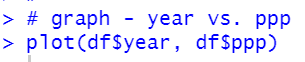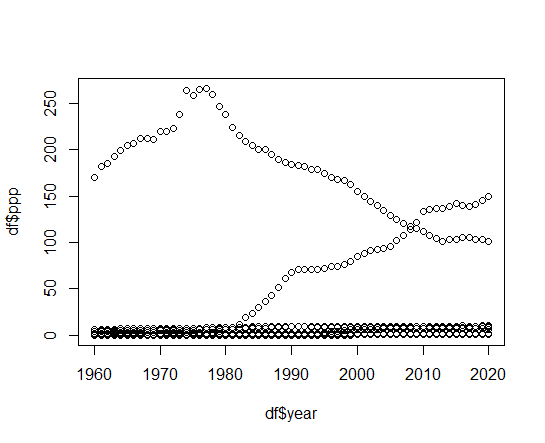We see 2 countries have relatively high ppp than oters.

Let's convert ppp to logarithmic value and make a graph.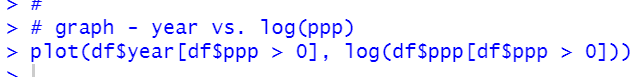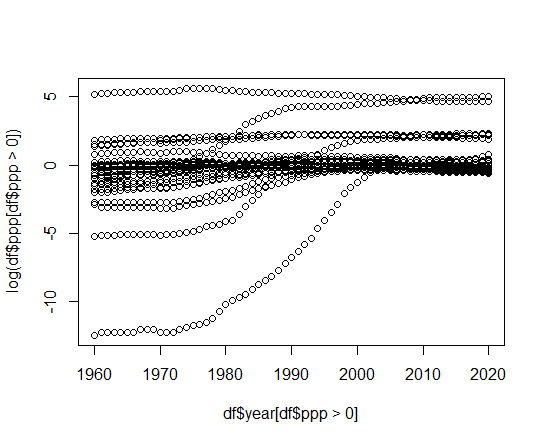We see 1960's ppp are more variance than 2020's ppp.

Let's make histogram. I use hist() function.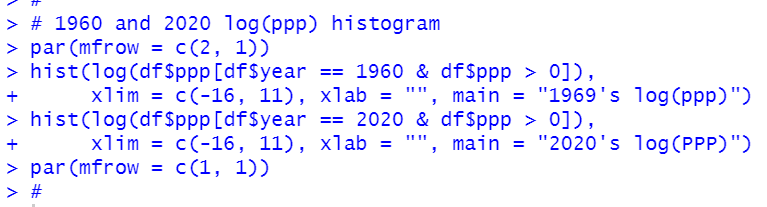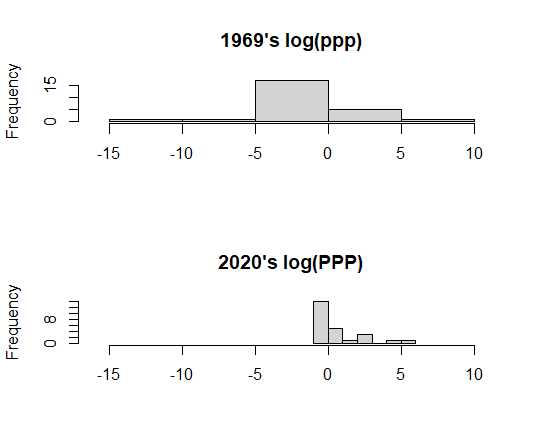Then, let's see iso vs. ppp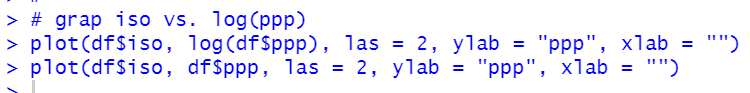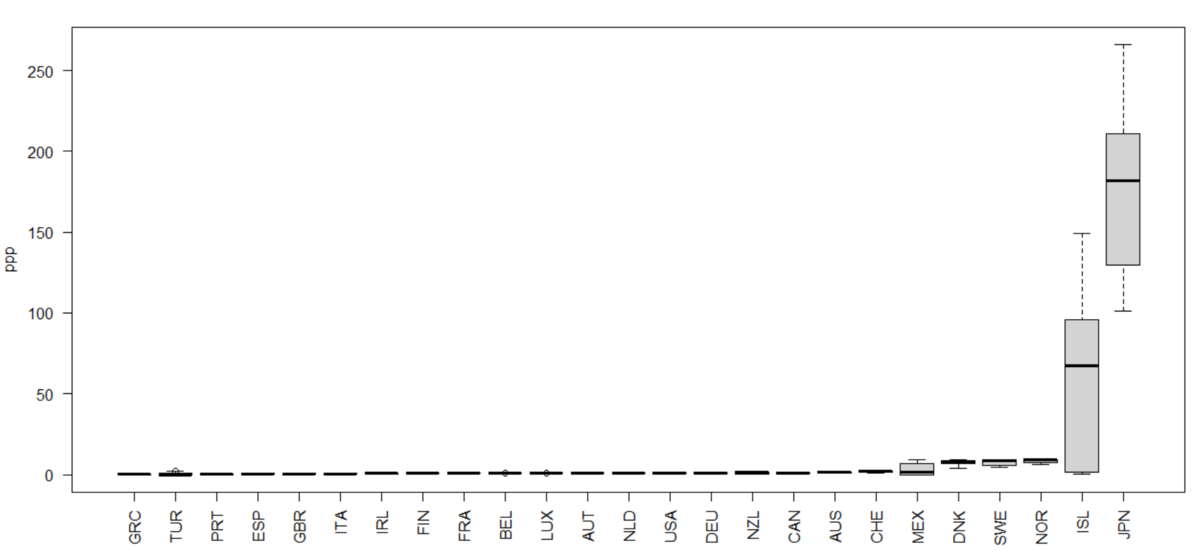I see ISL and JPN are very different than others.

Let's make a graph with log(ppp).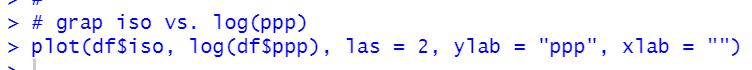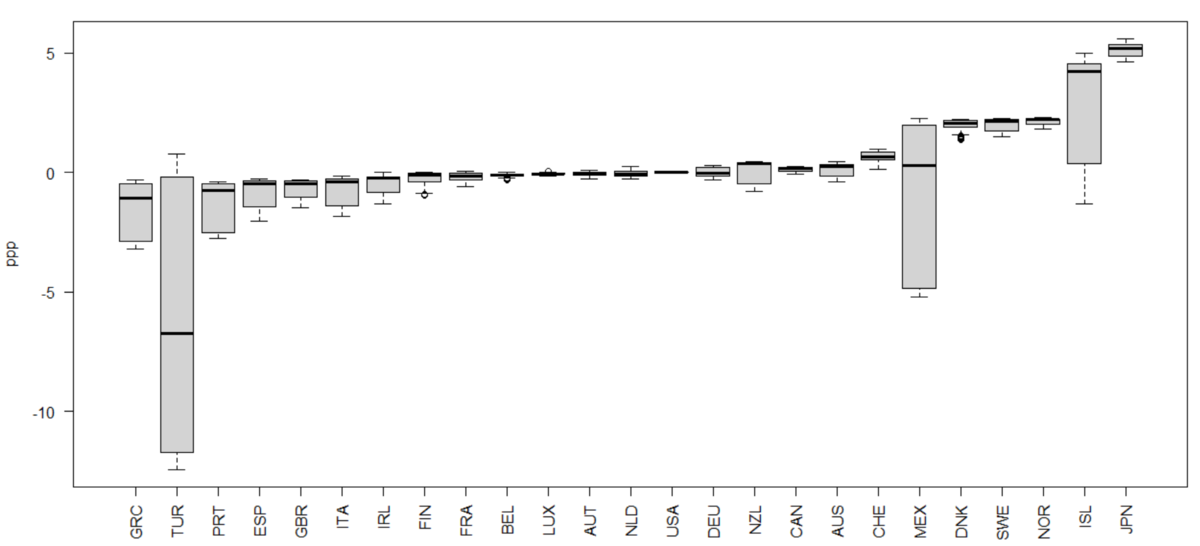I see TUR, MEX and ISL are very volatile.

Let's see which country are volatile/stable for ppp. I use filter(), group_by(), summarize() and arrange() function.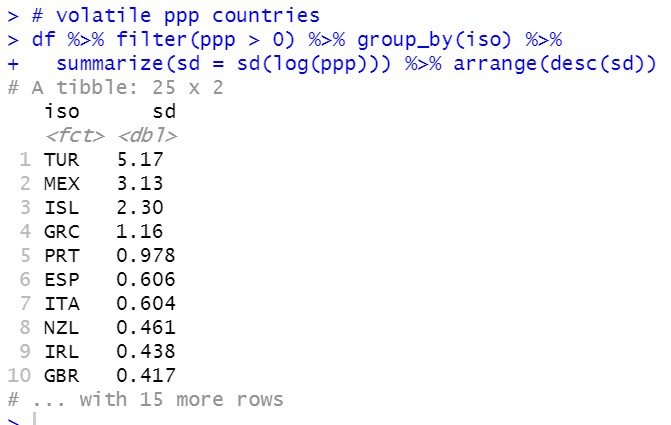As you see in the previous graph, TUR, MEAX and ISL are top 3 volatiole ppp countries.

Which are stable countries?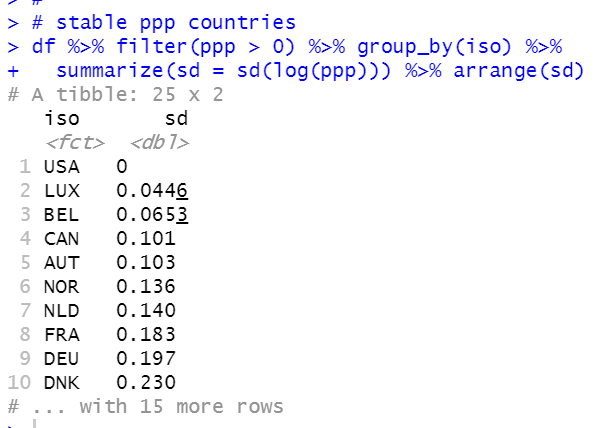USA has zero sd because ppp is based on us dollar. LUX, BEL and CAN are top 3 stable ppp countries.
Let's make a barchart. I use ggplot() + geom_bar() function and I use coord_flip() function to flip X-axis and Y-axis.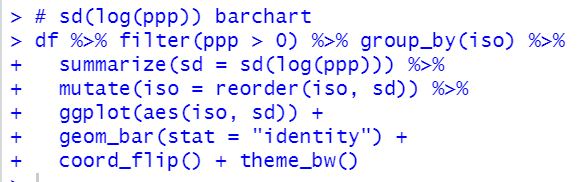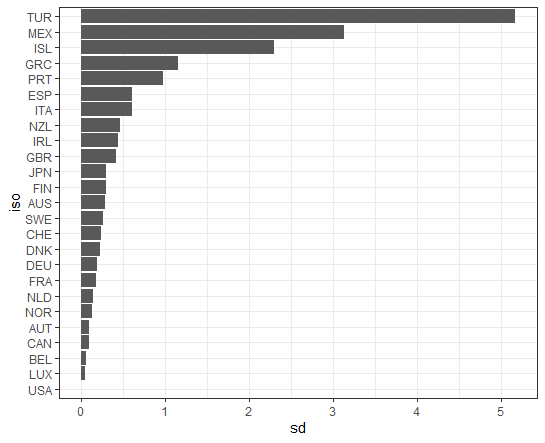That's it. Thank you!

Next post is